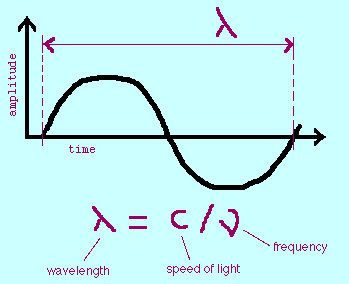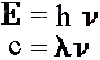# National Aeronautics and Space Administration

## Shedding a New Light on the Universe

### The Energetic Universe

Light has different colors because it has different energies. This is true whether we are talking about red and blue visible light, or infrared (IR) and X-ray light. Of all the colors in the visible spectrum, red light is the least energetic and blue is the most. Beyond the red end of the visible part of the spectrum lie infrared and radio light, both of which have lower energy than visible light. Above the blue end of the visible spectrum lies the higher energies of ultraviolet light, X-rays, and finally, gamma-rays

Because of light's unique properties, it can be described not only in terms of its energy, but also its wavelength, or its frequency. X-rays and gamma-rays are usually described in terms of energy, optical and infrared light in terms of wavelength, and radio in terms of frequency. This is a scientific convention that allows the use of the units that are the most convenient for describing whatever energy of light you are looking at. For example, it would be inconvenient to describe both low energy radio waves and high energy gamma-rays with the same units because the difference in their energies is so great. A radio wave can have an energy on the order of 4 x 10-10 eV as opposed to 4 x 109 eV for gamma-rays. That's an energy difference of 1019, or ten million trillion eV!Wavelength is the distance between two peaks of a wave, usually measured in meters (m). Frequency is the number of cycles of a wave to pass some point in a second. The units of frequency are thus cycles per second, or Hertz (Hz). Energy in astronomy is often measured in electron volts, or eV.

Wavelength and frequency are related by the speed of light (c), a fundamental constant, as shown by the animation above. Energy is also mathematically related to wavelength and frequency by Planck's constant (h). It was Max Planck who demonstrated that light sometimes behaves as a particle by showing that its energy (E), divided by its frequency (the Greek letter nu) is a constant. Since we know that frequency is equal to the speed of light (c) divided by wavelength (the Greek letter lambda), we also know the relationship between energy and wavelength.Since light can act like both a particle and a wave, we say that light has particle-wave duality. We call particles of light photons. Low-energy photons (i.e. radio) tend to behave more like waves, while higher energy photons (i.e. X-rays) behave more like particles. This is an important difference because it affects the way we build instruments to measure light.

Go to the Frequency, Wavelength and Energy Activity